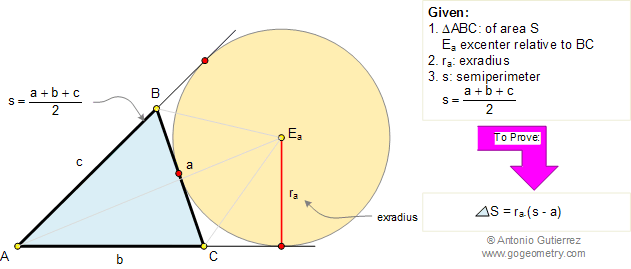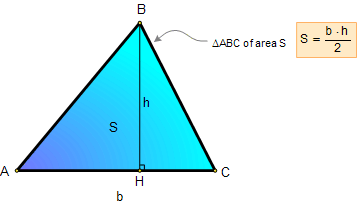Problem 194. Area of a Triangle, Semiperimeter, Exradius
 In the figure below, given a triangle ABC of area S, s is the semiperimeter (a + b + c) / 2, and ra is the exradius relative to side BC. Prove that S = ra.(s - a). View or post a solution1. AREA OF A TRIANGLE:

Proposition: The area of a triangle equals one-half the product of the length of a side and the length of the altitude to that side.Home | Geometry | Search | Problems | 191-200 | Semiperimeter | Area of a Triangle | Email | View or post a solution | By Antonio Gutierrez